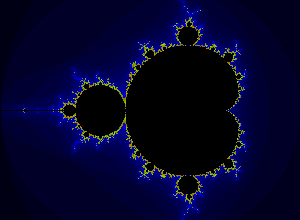# Making SVG fractals with perlI’ve just returned from visiting nor in Rotterdam. It being her birthday, we went to the pub with some of the lovely folks from her course, and the talk turned to SVG and if there was anything interesting you could do with it. I thought that maybe you could do something with fractals. In this I was probably recalling Mike’s awesome workshop on making fractals with HTML5 that happened at the 2011 barncamp. So, here's a first crack at a perl SVG Mandlebrot set maker. You can run it thus, you'll want to pipe the output to a .svg file most likely: ``` \$ perl fractal.pl -x-.5 -y-.7 -z1.5 > my.svg ``` Where

-x
Is the horizontal centre of the render.
-y
Is the vertical centre of the render.
-z
Is how far we are zoomed in.

You'll need perl installed and Readonly and Getopt::Std from the CPAN. At the moment, it is pitifully slow, probably an artifact of having to produce large amounts of XML. The HTML5 Canvas implementation that Mike did is better in just about every important way apart from making SVG. I might do a python version in the future, as that's the lingua franca of the folks on nor's course. But don't hold yer breath. ``` #!/usr/bin/perl use warnings; use strict; use Readonly; use Getopt::Std; Readonly my \$width => 300; # dimensions of svg to be produced Readonly my \$height => 220; Readonly my \$accuracy => 300; # higher looks better but takes longer Readonly my \$x_centre_default => -0.9; # horizontal and vertical centreing Readonly my \$y_centre_default => -0.4; Readonly my \$zoom_default => 0; # how far are we zoomed in to the fractal # option handling my %opts; getopt('xyz', \%opts); my \$x = \$opts{x} || \$x_centre_default; my \$y = \$opts{y} || \$y_centre_default; my \$z = \$opts{z} || \$zoom_default; # this is the main thing we do make_svg( \$x, \$y, \$z); # We'll make an array of colours for use by the make_svg sub sub make_colours { my @colours = ("rgb( 0, 0, 0 )"); for (1..99) { my ( \$red, \$green, \$blue ) = ( 0, 0, 0 ); if ( \$_ < 25) { \$red = 0; \$green = 0; \$blue = \$_ * 10; } elsif ( \$_ < 50 ) { \$red = 124; \$green = int( \$_/2 * 10); \$blue = 0; } else { \$red = int(\$_/4 * 10); \$green = 124; \$blue = 0; } push @colours, "rgb( \$red, \$green, \$blue )"; } return @colours; } # This makes a mandlebrot set. I am not a mathematician, though, so I may have miunderstood this. sub make_svg { my ( \$x_centre, \$y_centre, \$zoom ) = ( shift, shift, shift ); my @colours = make_colours; my \$svg = '<?xml version="1.0" standalone="no"?> <!DOCTYPE svg PUBLIC "-//W3C//DTD SVG 1.1//EN" "http://www.w3.org/Graphics/SVG/1.1/DTD/svg11.dtd">'; \$svg .= "\n<svg width=\"\$width\" height=\"\$height\" xmlns='http://www.w3.org/2000/svg' xmlns:xlink='http://www.w3.org/1999/xlink'>\n"; my \$z = 1; for( my \$k=0; \$k < \$zoom; \$k++ ){ \$z *= 10/3; } my \$zoomage = 0.6 * \$height * ( \$height/\$width ) * \$z; for ( my \$j = 0; \$j < \$height; \$j++ ) { for ( my \$i = 0; \$i < \$width; \$i++ ) { my \$x = 0; my \$y = 0; my \$foo = ( \$i - \$height / 2 ) / \$zoomage + \$x_centre; my \$bar = ( \$j - \$width / 2 ) / \$zoomage - \$y_centre; my \$iterations = 0; while ( ( \$x ** 2 + \$y ** 2 ) < 4.000000001 && \$iterations < \$accuracy ) { my \$xtmp = ( \$x ** 2 ) - ( \$y ** 2 ) + \$foo; \$y = 2 * \$x * \$y + \$bar; \$x = \$xtmp; \$iterations++; } if ( \$iterations >= \$accuracy ) { \$svg .= "\t<rect x='" . \$i . "' y='" . \$j . "' width='1' height='1' fill='" . \$colours . "'></rect>"; } else { my \$index = \$iterations % 99 + 1; \$svg .= "\t<rect x='" . \$i . "' y='" . \$j . "' width='1' height='1' fill='" . \$colours[\$index] . "'></rect>"; } \$svg .= "\n"; } } \$svg .= "</svg>\n"; print \$svg; } ```